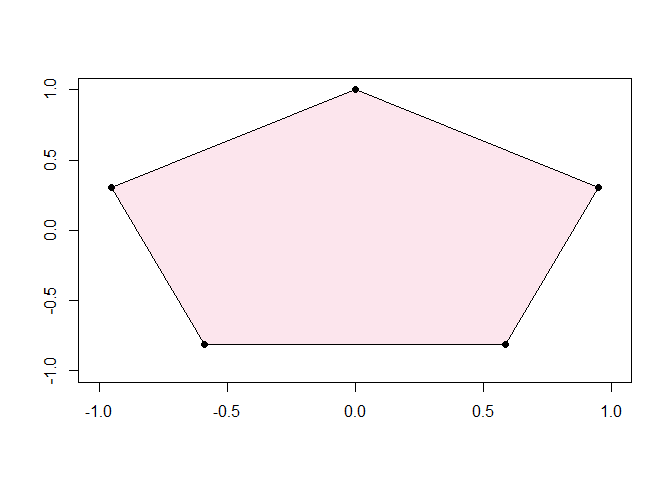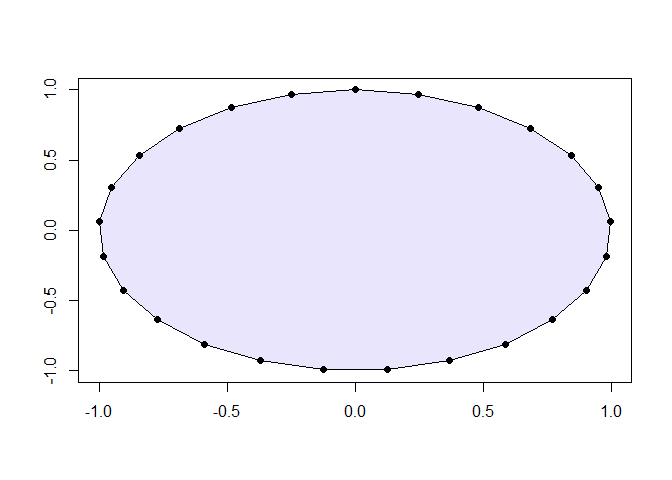## Functions in R

We have already seen and used quite a few of R’s built in functions:

function action
c() to create a vector
plot() to create a plot
which() to search a vector according to a condition
unique() to list the elements of a vector without repitition
length() to find the number of elements in a vector

We have also seen that functions take “arguments” which govern both to what, and how, the function is applied.

## Writing Functions in R

We write functions in R for a number of reasons:

a) R doesn’t have already have the function we need;

b) to avoid repetitive code;

c) to make code more efficient.

Writing bespoke functions is easy. Here’s the template to follow when writing your own functions:

name_of_function <- function(a.1, a.2, ..., a.n){

**instructions**

return(some result)

}  

Note the following:

a) give your function a memorable, verb-like name;

b) a.1, a.2, ..., a.n stand for the arguments the function will need;

c) the instructions are held between curly brackets { } and tell R what to do with the arguments;

d) return() is used to pass some result out of the function.

### example 1

A function to calculate the hypotenuse of a right angled triangle given the other two sides.

We all know the famous formula: $z = \sqrt{x^2 + y^2}.$ Our hypotenuse function will require two arguments: $$x$$ and $$y$$.

find.hypotenuse <- function(x, y){

h <- sqrt(x^2 + y^2)
return(h)

}

When you run this code, nothing will happen, R will simply read and learn your function. Let’s now call it:

find.hypotenuse(3, 4)
##  5

### example 2

A function to calculate the roots of a quadratic equation of the form:

$ax^2 + bx + c = 0$ We all know the famous formula: $x = \frac{-b \pm \sqrt{b^2 - 4ac}}{2a}$ Our quadratic solving function will need three arguments: $$a$$, $$b$$ and $$c$$.

quadsolv <- function(a,b,c){

D <- sqrt(b^2 - 4*a*c)

x1 <- (-b - D) / (2*a)
x2 <- (-b + D) / (2*a)

v <- c(x1, x2)

return(v)

}

Let’s use our new function to solve the quadratic equation

$x^2 - 7x + 6 = 0.$

quadsolv(1,-7, 6)
##  1 6

### example 3

A function to plot and shade a regular $$n$$-gon.

This function will have $$5$$ arguments; n, the number of sides required, r, g, b, numbers between $$0$$ and $$1$$ to define the mix of red, green and blue. Finally, t is a number between $$0$$ and $$1$$ which will control transparency. These last four arguments will be passed to a standard function called rgb() which can be called to handle colour mix.

Notice the insertion of comments using # in the code below. This is good practice so that you can return to your code later and understand what is going on!

draw.n.gon <- function(n, r, g, b, t){

N <- 1:n

#the following lines create n points evenly spread around a circle
#the +pi/2 ensures the polygon is the "right way up"

X <- cos(2*N*pi/n + (pi/2))
Y <- sin(2*N*pi/n + (pi/2))

#call for a null plot of appropriate size
plot(NULL, xlim = c(-1,1), ylim = c(-1,1), xlab = "", ylab = "")

#the colour is controlled by the red/green/blue/transparency mix rgb()
polygon(X,Y, col = rgb(r, g, b, t))

#finally the original points are added on top of the polygon
points(X,Y, pch = 16)

}
draw.n.gon(5,0.9,0,0.3,0.1)draw.n.gon(25,0.1,0,0.9,0.1)